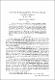## Solutions of the relativistic two-body problem. II. Quantum mechanics1972-04
CSIRO
##### Abstract
This paper discusses the formulation of a quantum mechanical equivalent of the relative time classical theory proposed in Part I. The relativistic wavefunction is derived and a covariant addition theorem is put forward which allows a covariant scattering theory to be established. The free particle eigenfunctions that are given are found not to be plane waves. A covariant partial wave analysis is also given. A means is described of converting wavefunctions that yield probability densities in 4-space to ones that yield the 3-space equivalents. Bound states are considered and covariant analogues of the Coulomb potential, harmonic oscillator potential, inverse cube law of force, square well potential, and two-body fermion interactions are discussed. © CSIRO 1972
##### Keywords
Quantum mechanics, Relativity theory, Scattering, Angular momentum, Bosons, Harmonic potential
##### Citation
Cook, J. L. (1972). Solutions of the relativistic two-body problem. II. Quantum mechanics. Australian Journal of Physics, 25(2), 141–146. doi:10.1071/PH720141